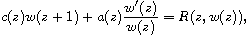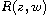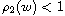Electron. J. Differential Equations, Vol. 2018 (2018), No. 93, pp. 1-12.

### Meromorphic solutions to non-linear differential-difference equations Changjiang Song, Kai Liu, Lei Ma

Abstract:
We consider the non-linear differential-difference equationwhere R(z,w(z)) is rational in w(z) with rational coefficients, a(z) and c(z) are non-zero rational functions. We give necessary conditions on the degree offor the above equation to admit a transcendental meromorphic solution of hyper-order. We also consider the admissible rational solutions of the above equation.

Submitted February 24, 2017. Published April 17, 2018.
Math Subject Classifications: 30D35, 39B32, 34M05.
Key Words: Meromorphic solutions; differential-difference equations; hyper-order.

Show me the PDF file (238 KB), TEX file for this article.Changjiang Song Department of Mathematics Nanchang University Nanchang, Jiangxi 330031, China email: Songchangjiang91@126.com Kai Liu Department of Mathematics Nanchang University Nanchang, Jiangxi 330031, China email: liukai418@126.com, liukai@ncu.edu.cn Lei Ma Department of Mathematics Nanchang University Nanchang, Jiangxi 330031, China email: malei92201@126.com